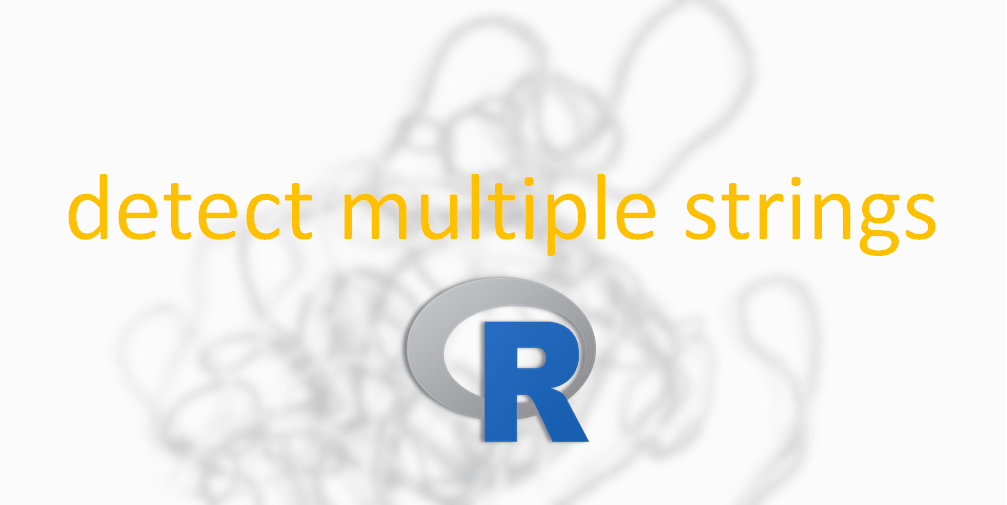# Detect combination of multiple strings in R

Here is how to detect multiple strings in R using base function grepl and alternatives, like str_detect from package stringr. There will be two main scenarios – detection of multiple strings simultaneously and any of the given strings.

## Detect combination of multiple strings at once in R

If you want to find out the appearance of multiple strings at once, then first and the simplest approach is a combination of multiple results of grepl. With two strings, it is not a lot of work but below is a more efficient approach that will be useful if there is a lot of text patterns.

```snames <- head(row.names(swiss))
snames

# "Courtelary"   "Delemont"     "Franches-Mnt" "Moutier"      "Neuveville"   "Porrentruy"

grepl("i", snames) & grepl("e", snames)

# FALSE FALSE FALSE  TRUE  TRUE FALSE```

If you want to get a logical vector with the result for each string appearance, you can use grepl in combination with sapply. As a result, you are getting a matrix of each pattern occurrence.

```p <- c("i", "e")

sapply(X = p, FUN = grepl, snames)

#         i    e
#[1,] FALSE TRUE
#[2,] FALSE TRUE
#[3,] FALSE TRUE
#[4,]  TRUE TRUE
#[5,]  TRUE TRUE
#[6,] FALSE TRUE```

You can use that row-wise with apply to detect multiple patterns simultaneously.

```apply(sapply(X = p, FUN = grepl, snames), MARGIN =  1, FUN = all)

# FALSE FALSE FALSE  TRUE  TRUE FALSE```

Here is another example of using apply row-wise.

### Detect combination of multiple strings at once in R with regex

If you use regex to detect a pattern in R, then you can get even more advantages. Here is an example that will check for the strings in a given order.

```snames <- head(row.names(swiss))
snames

# "Courtelary"   "Delemont"     "Franches-Mnt" "Moutier"      "Neuveville"   "Porrentruy"

grepl("(.*a)(.*e)", snames, perl = TRUE)

# FALSE FALSE  TRUE FALSE FALSE FALSE```

If you want to produce results of an AND operator in regex, then here is how to do that.

```grepl("(?=.*i)(?=.*e)", snames, perl = TRUE)

# FALSE FALSE FALSE  TRUE  TRUE FALSE```

## Detect one of the multiple strings in R

Occurrence detection of one of the strings is a little bit easier. You can work with the multiple grepl functions.

```snames <- head(row.names(swiss))
snames

# "Courtelary"   "Delemont"     "Franches-Mnt" "Moutier"      "Neuveville"   "Porrentruy"

grepl("i", snames) | grepl("o", snames)

#  TRUE  TRUE FALSE  TRUE  TRUE  TRUE```

If you have a lot of patterns that you want to check, then it is better to use grepl with sapply and apply functions.

```p <- c("i", "o")

apply(sapply(X = p, FUN = grepl, snames), MARGIN =  1, FUN = any)

#  TRUE  TRUE FALSE  TRUE  TRUE  TRUE```

Another approach in the detection of any of the strings is the usage of the OR operator in regex. Be careful and do not use extra space symbols with the vertical pipe.

```grepl(paste(p, collapse = "|"), snames)

#  TRUE  TRUE FALSE  TRUE  TRUE  TRUE```

Here is another example where you can use this technique to extract text based on the occurrence of multiple strings.

## Alternatives of grepl

One of the most popular alternatives of grepl is the str_detect function from stringr.
Besides stringr, there is also a package stringi that is very popular with similar function names. That might be confusing.

If you would like to understand the difference between them, please check out this post. There is a good comparison and comments on the content of each package.

I did not found str_detect very different from grepl most of the time.

Here is the detection of simultaneously appearing strings with str_detect.

```snames <- head(row.names(swiss))
snames

# "Courtelary"   "Delemont"     "Franches-Mnt" "Moutier"      "Neuveville"   "Porrentruy"

stringr::str_detect(snames, "(?=.*i)(?=.*e)")

# FALSE FALSE FALSE  TRUE  TRUE FALSE```

Here is an appearance detection of any strings with str_detect.

```p <- c("i", "o")

stringr::str_detect(snames, paste(p, collapse = "|"))

#  TRUE  TRUE FALSE  TRUE  TRUE  TRUE```

If you are working with data processing using pipes in R, then it is a little bit easier to use str_detect instead of grepl.

```require(dplyr)

snames %>% stringr::str_detect(paste(p, collapse = "|"))

snames %>% grepl(paste(p, collapse = "|"), .)```

If you are working with pipes in R, check out my top 10 favorite dplyr tips and tricks.

Categories R

### 1 comment on “Detect combination of multiple strings in R”

1.mdidish

You can also use grepl(“i|o”, snames).